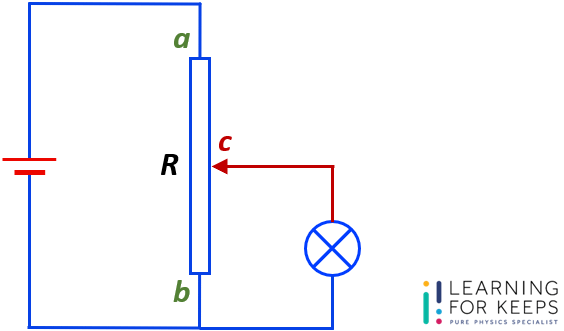# Question Guide – DC Circuit – Resistance of a Potential Divider Circuit

Question:
The figure shows a circuit that uses a potential divider R.The resistance of R is 10 Ω.
R is divided into 2 sections, ac and bc, by the slider c.
Section ac of R has a resistance of 3.2 Ω.
The resistance of the lamp is also 3.2 Ω.
Calculate the total resistance of the circuit.

Solutions are open to Learning For Keeps students who are preparing for the upcoming O Level exam only.